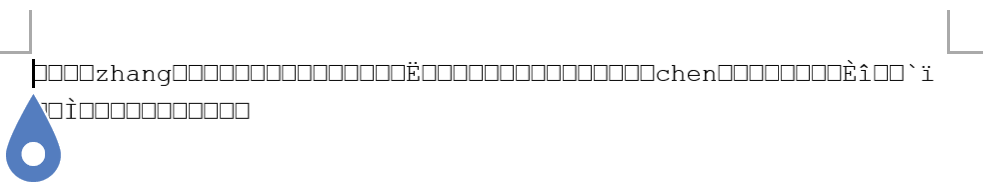c语言把结构体内容写入文件问题

#include
#define m 2
main(){
int i;
FILE *fp;
typedef struct{
int year;
int month;
int day;
}date;
struct student{
int number;

char name;
date birth;
}student1[m];
fp=fopen("student.doc","w");
for(i=0;i<m;i++){
student1[i].number=i;
scanf("%s",student1[i].name);
scanf("%d%d%d",&(student1[i].birth.year),&(student1[i].birth.month),&(student1[i].birth.day));
fwrite(&(student1[i]),sizeof(struct student),1,fp);
}
fclose(fp);
}4个回答

fp = fopen("student.doc", "w");这句不对，写入doc不能用fopen3 年多之前 回复3 年多之前 回复

fp=fopen("student.doc","w");
for(i=0;i<m;i++){
student1[i].number=i;
scanf("%s",student1[i].name);
scanf("%d%d%d",&(student1[i].birth.year),&(student1[i].birth.month),&(student1[i].birth.day));
fwrite(&(student1[i]),sizeof(struct student),1,fp);
}
fclose(fp);

fp=fopen("student.doc","w");
fwrite(&(student1[i]),sizeof(struct student),m,fp);
}
fclose(fp);

`````` #include<stdio.h>
#define m  2
void main(){
int  i;
FILE  *fp;
typedef struct{
int     year;
int     month;
int      day;
}date;
struct    student{
int        number;
char    name;
date     birth;
}student1[m];
fp = fopen("student.txt", "w");
for (i = 0; i<m; i++){
student1[i].number = i;
scanf("%s", student1[i].name);
scanf("%d%d%d", &(student1[i].birth.year), &(student1[i].birth.month), &(student1[i].birth.day));
fwrite(&(student1[i]), sizeof(struct student), 1, fp);
}
fclose(fp);

struct student student2[m];
fp = fopen("student.txt", "r");
for (i = 0; i<m; i++){
printf("%s%d%d%d\n", student2[i].name,(student2[i].birth.year), (student2[i].birth.month), (student2[i].birth.day));

}
fclose(fp);

}
``````

bzero memset刷新一下，c++和c语言的结构体是不是兼容的

C语言 带结构体的链表（如补充所示） 如何创建？

c语言如何把链表数据写入文件？

C语言中，有没有办法让数组（结构体）数据保存到一个文本文档或者是其他的什么的里面

C语言结构顺序表数据读写问题

```c #include<stdio.h> int main(void) { struct message { char sb; char name; int score; }; int n; int i; scanf("%d",&n); struct message stu[n]; for(i=0;i<n;i++) { scanf("%s %s %d",stu[i].sb,stu[i].name,&stu[i].score); printf("%s %s\n",stu[i].name,stu[i].sb); } return 0; } ``` ![图片说明](https://img-ask.csdn.net/upload/201906/05/1559734658_370024.jpg) 结果和数组大小有关系，这是什么情况？，求大佬解答（卑微）

C语言,从文件中读取数据给结构体变量赋值,不知道哪里错了

#include <stdio.h> #include <stdlib.h> #include <string.h> struct student { int number; }num; int main() { struct student s1, *s2; s2 = &s1; FILE *fp = fopen("test.txt", "r"); fscanf(fp, "%d", s2->number); return 0; }![图片说明](https://img-ask.csdn.net/upload/201603/10/1457580600_112754.png)

c语言读写文件时出现的问题

C语言写入数据到二进制文件失败

# 相关代码如下 # 我在add（）调用lessondatewrite（）写进去了 #但是lessondateltater（）调用lessondatewrite（）没写进去 # 调试结果![图片说明](https://img-ask.csdn.net/upload/201811/02/1541169947_50238.png) #运行结果 英语行的 40 应该变成39 ![图片说明](https://img-ask.csdn.net/upload/201811/02/1541169992_687847.png) ``` typedef struct //定义一个Lesson类型的结构体 { char name; char techer; char location; char time; int number; }LESSON; /*课程结构体参数 课程名 char 50字节 授课老师 char 50字节 位置 char 50字节 开课时间 char 50字节 座位数 int 4字节 结构体大小总计 204字节*/ LESSON lessondatewrite(FILE *fl,LESSON date)//这是一个结构体类型的函数，它可以写入课程数据，接受一个文件指针 { fflush(fl);//刷新缓存区 使将要输入的数据写入硬盘 一次性输入大量数据时使用 避免输入失败 fwrite(&date,sizeof(LESSON),1,fl); } void add()//添加一个课程数据 { FILE *lessondate; LESSON lesson={0};// 声明一个LESSON类型的变量为lesson if((lessondate=fopen("lessondate","rb+"))==NULL)//若文件不存在，则建立文件 { lessondate=fopen("lessondate","wb+"); //rb+打开二进制文本文件，允许读写，若不存在返回空 //wb+新建二进制文本文件，允许读写。如果文件存在则删除文件重建 } printf("请依次输入如下数据，课程名，授课老师，教室位置，开课时间（xx月xx日xx:xx），座位数（各数据以空格隔开，回车结束）\n"); scanf("%s %s %s %s %d",&lesson.name,&lesson.techer,&lesson.location,&lesson.time,&lesson.number); //scanf在接收字符串时 不会接收空白符（空格与回车） 逗号或其他都能接收 //%s不能使用char 而是char[] fseek(lessondate,0L,SEEK_END); lessondatewrite(lessondate,lesson); fclose(lessondate); system("cls"); admin(); } void lessondatelater(int choose) { FILE *lessondate; LESSON lesson={0}; int i; lessondate=fopen("lessondate","rb+"); for(i=0;i<choose;i++) { lesson=lessondateread(lessondate);//给读取函数传递一个文件指针 假定有是三个结构体A B C 循环结束后光标在C前面B后面 } fseek(lessondate,-204L,SEEK_CUR);//后退到上一个结构体 此时光标在B前面 lesson=lessondateread(lessondate);//读取这个结构体数据 读取B的数据 此时光标前进到C前面 lesson.number--;//修改结构体数据 fseek(lessondate,-204L,SEEK_CUR);//后退到上一个结构体 此时光标在B前面 lessondatewrite(lessondate,lesson);//写入修改后结构体数据 覆盖B } ```

#include<stdio.h> #include<stdlib.h> struct Student { char name; int num; int age; char addr; }stud; void Save() { FILE *fp; int i; if((fp=fopen("stu.txt","wb"))==NULL) { printf("cann't open file"); return; } for(i=0;i<2;i++) if(fwrite(&stud[i],sizeof(struct Student),1,fp)!=1) printf("file write error\n"); fclose(fp); } int main() { int i; printf("Please enter data of student:\n"); for(i=0;i<2;i++) scanf("%s%d%d%s",&stud[i].name,&stud[i].num,&stud[i].age,&stud[i].addr); Save(); return 0; } ![图片说明](https://img-ask.csdn.net/upload/201806/07/1528372246_877736.png)

C语言 结构体指针字符 二进制文件写入和读取 指针字符输出错误

typedef struct s{ char *name; }student; /*将结构体读出并显示*/ int IsUsed(char *str) { FILE *fp = fopen("test","rb"); student s; s.name = (char*) malloc(sizeof(char)*20); fread(&s,sizeof(student),1,fp); while(!feof(fp)){ printf("%s",s.name); //为什么这里出错？？？？？？？？？？？？？？？？？？ if(strcmp(s.name,str)==0){ fclose(fp); return 1; //存在该字符串，则返回1 } fread(&s,sizeof(student),1,fp); } fclose(fp); return 0; //不存在该字符串，则返回0 } int main() { FILE *fp = fopen("test","rb+"); /*先将结构体P存入二进制文件中*/ student p; p.name = (char*) malloc(sizeof(char)*20); scanf("%s",p.name); while(IsUsed(p.name)){ //检查员工号是否唯一 printf("该字符串已经存在，请更换一个："); scanf("%s",p.name); } fwrite(&p,sizeof(student),1,fp); } 在printf哪里显示程序停止工作！！！！！！！！！求解啊

c语言中怎样将数值型数组写到txt文件中，并且打开文件时不是一堆乱码？

Vue + Spring Boot 项目实战（十九）：Web 项目优化解决方案

CopyOnWriteArrayList.java和ArrayList.java，这2个类的构造函数，注释中有一句话 1 2 3 4 5 6 7 8 9 10 11 12 13 14 15 public ArrayList(Collection&lt;? ...

CSDN：因博主近期注重写专栏文章(已超过150篇)，订阅博主专栏人数在突增，近期很有可能提高专栏价格(已订阅的不受影响)，提前声明，敬请理解！

CSDN：因博主近期注重写专栏文章(已超过150篇)，订阅博主专栏人数在突增，近期很有可能提高专栏价格(已订阅的不受影响)，提前声明，敬请理解！ 目录 博客声明 大数据了解博主粉丝 博主的粉丝群体画像 粉丝群体性别比例、年龄分布 粉丝群体学历分布、职业分布、行业分布 国内、国外粉丝群体地域分布 博主的近期访问每日增量、粉丝每日增量 博客声明 因近期博主写专栏的文章越来越多，也越来越精细，逐步优化文章。因此，最近一段时间，订阅博主专栏的人数增长也非常快，并且专栏价

HashMap底层实现原理，红黑树，B+树，B树的结构原理 Spring的AOP和IOC是什么？它们常见的使用场景有哪些？Spring事务，事务的属性，传播行为，数据库隔离级别 Spring和SpringMVC，MyBatis以及SpringBoot的注解分别有哪些？SpringMVC的工作原理，SpringBoot框架的优点，MyBatis框架的优点 SpringCould组件有哪些，他们...

97年世界黑客编程大赛冠军作品（大小仅为16KB），惊艳世界的编程巨作

A站 AcFun弹幕视频网，简称“A站”，成立于2007年6月，取意于Anime Comic Fun，是中国大陆第一家弹幕视频网站。A站以视频为载体，逐步发展出基于原生内容二次创作的完整生态，拥有高质量互动弹幕，是中国弹幕文化的发源地；拥有大量超粘性的用户群体，产生输出了金坷垃、鬼畜全明星、我的滑板鞋、小苹果等大量网络流行文化，也是中国二次元文化的发源地。 B站 全称“哔哩哔哩(bilibili...

Java14 新特性解读

Java14 已于 2020 年 3 月 17 号发布，官方特性解读在这里：https://openjdk.java.net/projects/jdk/14/以下是个人对于特性的中文式...